# Inheritance in Java

• Difficulty Level : Easy
• Last Updated : 25 May, 2022

Inheritance is an important pillar of OOP(Object-Oriented Programming). It is the mechanism in java by which one class is allowed to inherit the features(fields and methods) of another class.Important terminology:

• Super Class: The class whose features are inherited is known as superclass(or a base class or a parent class).
• Sub Class: The class that inherits the other class is known as a subclass(or a derived class, extended class, or child class). The subclass can add its own fields and methods in addition to the superclass fields and methods.
• Reusability: Inheritance supports the concept of “reusability”, i.e. when we want to create a new class and there is already a class that includes some of the code that we want, we can derive our new class from the existing class. By doing this, we are reusing the fields and methods of the existing class.

How to use inheritance in Java

The keyword used for inheritance is extends

Syntax :

```class derived-class extends base-class
{
//methods and fields
}  ```

Example: In the below example of inheritance, class Bicycle is a base class, class MountainBike is a derived class that extends Bicycle class and class Test is a driver class to run program.

## Java

 `// Java program to illustrate the ` `// concept of inheritance ` ` `  `// base class ` `class` `Bicycle { ` `    ``// the Bicycle class has two fields ` `    ``public` `int` `gear; ` `    ``public` `int` `speed; ` ` `  `    ``// the Bicycle class has one constructor ` `    ``public` `Bicycle(``int` `gear, ``int` `speed) ` `    ``{ ` `        ``this``.gear = gear; ` `        ``this``.speed = speed; ` `    ``} ` ` `  `    ``// the Bicycle class has three methods ` `    ``public` `void` `applyBrake(``int` `decrement) ` `    ``{ ` `        ``speed -= decrement; ` `    ``} ` ` `  `    ``public` `void` `speedUp(``int` `increment) ` `    ``{ ` `        ``speed += increment; ` `    ``} ` ` `  `    ``// toString() method to print info of Bicycle ` `    ``public` `String toString() ` `    ``{ ` `        ``return` `(``"No of gears are "` `+ gear + ``"\n"` `                ``+ ``"speed of bicycle is "` `+ speed); ` `    ``} ` `} ` ` `  `// derived class ` `class` `MountainBike ``extends` `Bicycle { ` ` `  `    ``// the MountainBike subclass adds one more field ` `    ``public` `int` `seatHeight; ` ` `  `    ``// the MountainBike subclass has one constructor ` `    ``public` `MountainBike(``int` `gear, ``int` `speed, ` `                        ``int` `startHeight) ` `    ``{ ` `        ``// invoking base-class(Bicycle) constructor ` `        ``super``(gear, speed); ` `        ``seatHeight = startHeight; ` `    ``} ` ` `  `    ``// the MountainBike subclass adds one more method ` `    ``public` `void` `setHeight(``int` `newValue) ` `    ``{ ` `        ``seatHeight = newValue; ` `    ``} ` ` `  `    ``// overriding toString() method ` `    ``// of Bicycle to print more info ` `    ``@Override` `public` `String toString() ` `    ``{ ` `        ``return` `(``super``.toString() + ``"\nseat height is "` `                ``+ seatHeight); ` `    ``} ` `} ` ` `  `// driver class ` `public` `class` `Test { ` `    ``public` `static` `void` `main(String args[]) ` `    ``{ ` ` `  `        ``MountainBike mb = ``new` `MountainBike(``3``, ``100``, ``25``); ` `        ``System.out.println(mb.toString()); ` `    ``} ` `}`

Output

```No of gears are 3
speed of bicycle is 100
seat height is 25
```

In the above program, when an object of MountainBike class is created, a copy of all methods and fields of the superclass acquire memory in this object. That is why by using the object of the subclass we can also access the members of a superclass.

Please note that during inheritance only the object of the subclass is created, not the superclass. For more, refer Java Object Creation of Inherited Class

Illustrative image of the program: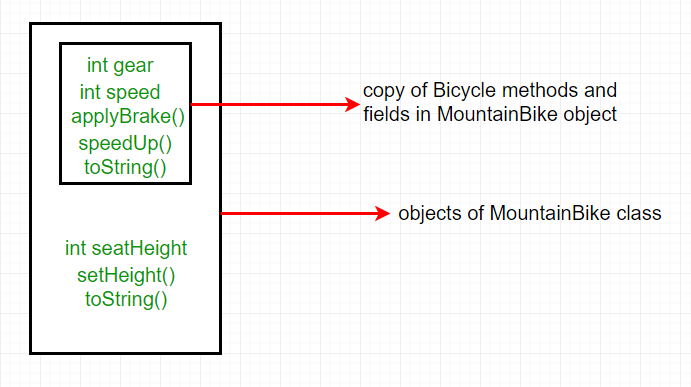In practice, inheritance and polymorphism are used together in java to achieve fast performance and readability of code.

Types of Inheritance in Java

Below are the different types of inheritance which are supported by Java.

1. Single Inheritance: In single inheritance, subclasses inherit the features of one superclass. In the image below, class A serves as a base class for the derived class B.

## Java

 `// Java program to illustrate the ` `// concept of single inheritance ` `import` `java.io.*; ` `import` `java.lang.*; ` `import` `java.util.*; ` ` `  `class` `one { ` `    ``public` `void` `print_geek() ` `    ``{ ` `        ``System.out.println(``"Geeks"``); ` `    ``} ` `} ` ` `  `class` `two ``extends` `one { ` `    ``public` `void` `print_for() { System.out.println(``"for"``); } ` `} ` `// Driver class ` `public` `class` `Main { ` `    ``public` `static` `void` `main(String[] args) ` `    ``{ ` `        ``two g = ``new` `two(); ` `        ``g.print_geek(); ` `        ``g.print_for(); ` `        ``g.print_geek(); ` `    ``} ` `}`

Output

```Geeks
for
Geeks
```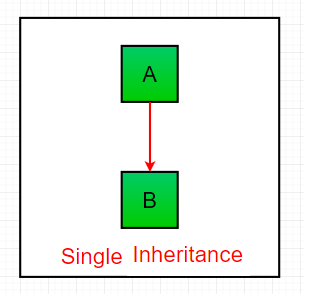2. Multilevel Inheritance: In Multilevel Inheritance, a derived class will be inheriting a base class and as well as the derived class also act as the base class to other class. In the below image, class A serves as a base class for the derived class B, which in turn serves as a base class for the derived class C. In Java, a class cannot directly access the grandparent’s members.

## Java

 `// Java program to illustrate the ` `// concept of Multilevel inheritance ` `import` `java.io.*; ` `import` `java.lang.*; ` `import` `java.util.*; ` ` `  `class` `one { ` `    ``public` `void` `print_geek() ` `    ``{ ` `        ``System.out.println(``"Geeks"``); ` `    ``} ` `} ` ` `  `class` `two ``extends` `one { ` `    ``public` `void` `print_for() { System.out.println(``"for"``); } ` `} ` ` `  `class` `three ``extends` `two { ` `    ``public` `void` `print_geek() ` `    ``{ ` `        ``System.out.println(``"Geeks"``); ` `    ``} ` `} ` ` `  `// Drived class ` `public` `class` `Main { ` `    ``public` `static` `void` `main(String[] args) ` `    ``{ ` `        ``three g = ``new` `three(); ` `        ``g.print_geek(); ` `        ``g.print_for(); ` `        ``g.print_geek(); ` `    ``} ` `}`

Output

```Geeks
for
Geeks
```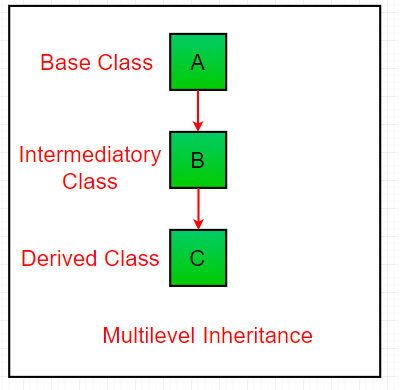3. Hierarchical Inheritance: In Hierarchical Inheritance, one class serves as a superclass (base class) for more than one subclass. In the below image, class A serves as a base class for the derived class B, C and D.

## Java

 `// Java program to illustrate the ` `// concept of Hierarchical  inheritance ` ` `  `class` `A { ` `    ``public` `void` `print_A() { System.out.println(``"Class A"``); } ` `} ` ` `  `class` `B ``extends` `A { ` `    ``public` `void` `print_B() { System.out.println(``"Class B"``); } ` `} ` ` `  `class` `C ``extends` `A { ` `    ``public` `void` `print_C() { System.out.println(``"Class C"``); } ` `} ` ` `  `class` `D ``extends` `A { ` `    ``public` `void` `print_D() { System.out.println(``"Class D"``); } ` `} ` ` `  `// Driver Class ` `public` `class` `Test { ` `    ``public` `static` `void` `main(String[] args) ` `    ``{ ` `        ``B obj_B = ``new` `B(); ` `        ``obj_B.print_A(); ` `        ``obj_B.print_B(); ` ` `  `        ``C obj_C = ``new` `C(); ` `        ``obj_C.print_A(); ` `        ``obj_C.print_C(); ` ` `  `        ``D obj_D = ``new` `D(); ` `        ``obj_D.print_A(); ` `        ``obj_D.print_D(); ` `    ``} ` `}`

Output

```Class A
Class B
Class A
Class C
Class A
Class D
```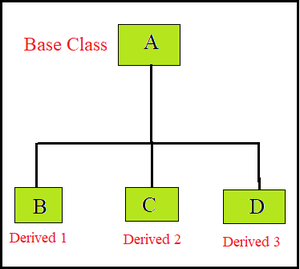Hierarchical Inheritance

4. Multiple Inheritance (Through Interfaces): In Multiple inheritances, one class can have more than one superclass and inherit features from all parent classes. Please note that Java does not support multiple inheritances with classes. In java, we can achieve multiple inheritances only through Interfaces. In the image below, Class C is derived from interface A and B.

## Java

 `// Java program to illustrate the ` `// concept of Multiple inheritance ` `import` `java.io.*; ` `import` `java.lang.*; ` `import` `java.util.*; ` ` `  `interface` `one { ` `    ``public` `void` `print_geek(); ` `} ` ` `  `interface` `two { ` `    ``public` `void` `print_for(); ` `} ` ` `  `interface` `three ``extends` `one, two { ` `    ``public` `void` `print_geek(); ` `} ` `class` `child ``implements` `three { ` `    ``@Override` `public` `void` `print_geek() ` `    ``{ ` `        ``System.out.println(``"Geeks"``); ` `    ``} ` ` `  `    ``public` `void` `print_for() { System.out.println(``"for"``); } ` `} ` ` `  `// Drived class ` `public` `class` `Main { ` `    ``public` `static` `void` `main(String[] args) ` `    ``{ ` `        ``child c = ``new` `child(); ` `        ``c.print_geek(); ` `        ``c.print_for(); ` `        ``c.print_geek(); ` `    ``} ` `}`

Output

```Geeks
for
Geeks
```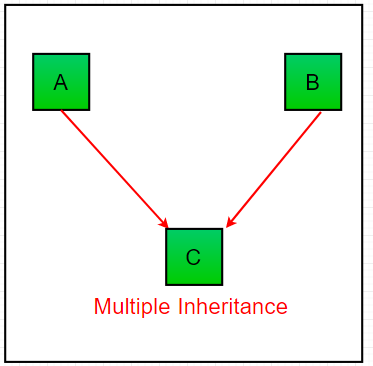5. Hybrid Inheritance(Through Interfaces): It is a mix of two or more of the above types of inheritance. Since java doesn’t support multiple inheritances with classes, hybrid inheritance is also not possible with classes. In java, we can achieve hybrid inheritance only through Interfaces.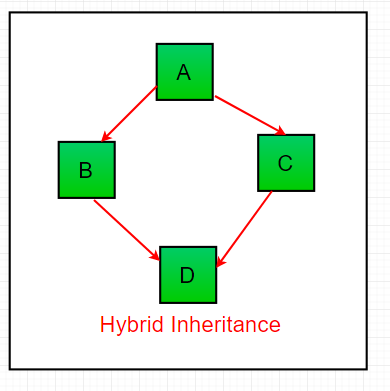Important facts about inheritance in Java

• Default superclass: Except Object class, which has no superclass, every class has one and only one direct superclass (single inheritance). In the absence of any other explicit superclass, every class is implicitly a subclass of the Object class.
• Superclass can only be one: A superclass can have any number of subclasses. But a subclass can have only one superclass. This is because Java does not support multiple inheritances with classes. Although with interfaces, multiple inheritances are supported by java.
• Inheriting Constructors: A subclass inherits all the members (fields, methods, and nested classes) from its superclass. Constructors are not members, so they are not inherited by subclasses, but the constructor of the superclass can be invoked from the subclass.
• Private member inheritance: A subclass does not inherit the private members of its parent class. However, if the superclass has public or protected methods(like getters and setters) for accessing its private fields, these can also be used by the subclass.

Java IS-A type of Relationship.

IS-A is a way of saying: This object is a type of that object. Let us see how the extends keyword is used to achieve inheritance.

## Java

 `public` `class` `SolarSystem { ` `} ` `public` `class` `Earth ``extends` `SolarSystem { ` `} ` `public` `class` `Mars ``extends` `SolarSystem { ` `} ` `public` `class` `Moon ``extends` `Earth { ` `}`

Now, based on the above example, in Object-Oriented terms, the following are true:-

1. SolarSystem the superclass of Earth class.
2. SolarSystem the superclass of Mars class.
3. Earth and Mars are subclasses of SolarSystem class.
4. Moon is the subclass of both Earth and SolarSystem classes.

## Java

 `class` `SolarSystem { ` `} ` `class` `Earth ``extends` `SolarSystem { ` `} ` `class` `Mars ``extends` `SolarSystem { ` `} ` `public` `class` `Moon ``extends` `Earth { ` `    ``public` `static` `void` `main(String args[]) ` `    ``{ ` `        ``SolarSystem s = ``new` `SolarSystem(); ` `        ``Earth e = ``new` `Earth(); ` `        ``Mars m = ``new` `Mars(); ` ` `  `        ``System.out.println(s ``instanceof` `SolarSystem); ` `        ``System.out.println(e ``instanceof` `Earth); ` `        ``System.out.println(m ``instanceof` `SolarSystem); ` `    ``} ` `}`

Output

```true
true
true
```

What all can be done in a Subclass?

In sub-classes we can inherit members as is, replace them, hide them, or supplement them with new members:

• The inherited fields can be used directly, just like any other fields.
• We can declare new fields in the subclass that are not in the superclass.
• The inherited methods can be used directly as they are.
• We can write a new instance method in the subclass that has the same signature as the one in the superclass, thus overriding it (as in the example above, toString() method is overridden).
• We can write a new static method in the subclass that has the same signature as the one in the superclass, thus hiding it.
• We can declare new methods in the subclass that are not in the superclass.
• We can write a subclass constructor that invokes the constructor of the superclass, either implicitly or by using the keyword super.

My Personal Notes arrow_drop_up
Recommended Articles
Page :